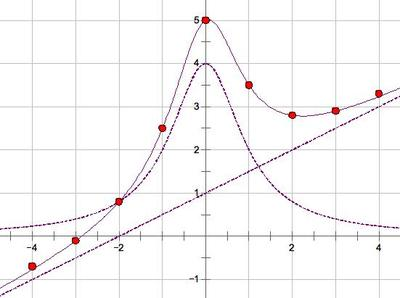# A Sum of Functions

Alignments to Content Standards: F-BF.A.1.b F-BF.A.1

Using the graphs below, sketch a graph of the function $s(x) = f(x) + g(x)$.## IM Commentary

The intent of this problem is to have students think about how function addition works on a fundamental level, so formulas have been omitted on purpose. In the graph shown, $g(x)=\frac{4}{x^2+1}$. The task may be extended by asking students to sketch the graph of $d(x)=f(x)-g(x)$.

Although this problem does not ask students to "write a function that describes a relationship between two quantities", it can provide students with understandings preparatory for F.BF.1b. In addition, this task makes use of the reasoning required for F.BF.3.

Source: Hilton Russell

## Solutions

Solution: Graphical solution

Students can create the graph shown below by:

1. visually estimating the distance between the graph of $f$ and the $x$-axis at a particular integer value of $x$, and
2. plotting a point this distance above (or below, if the $f(x)$ value is negative) the graph of $g$.

Some students may want to use a strip of paper to mark a distance and then use the mark to help them plot the point.Solution: Numerical solution

Students may also create a chart of approximate values of $f(x)$ and $g(x)$ at various $x$-values by estimating from the provided graphs. We then add a row of $s(x)$ values by summing the two rows above. Finally, we plot points of the form $(x, s(x))$ to sketch the graph of $y=s(x)$.

 $x$ -4 -3 -2 -1 0 1 2 3 4 $f(x)\approx$ -1 -0.5 0 0.5 1 1.5 2 2.5 3 $g(x)\approx$ 0.2 0.4 0.8 2 4 2 0.8 0.4 0.2 $s(x)=f(x) + g(x)\approx$ -0.8 -0.1 0.8 2.5 5 3.5 2.8 2.9 3.2